Next: Combining the two models Up: Bayesian nonlinear state-space model Previous: The prior of the   Contents

## The approximating posterior distribution

As with the HMM, the approximating posterior distribution is chosen to have a factorial form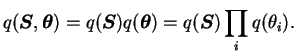(5.41)

The independent distributions for the parameters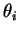are all Gaussian with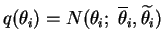(5.42)

where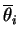and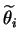are the variational parameters whose values must be optimised to minimise the cost function.

Because of the strong temporal correlations between the source values at consecutive time instants, the same approach cannot be applied to form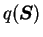. Therefore the approximation is chosen to be of the form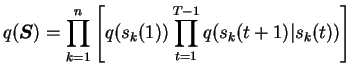(5.43)

where the factors are again Gaussian.

The distributions for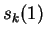can be handled as before with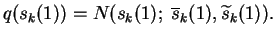(5.44)

The conditional distribution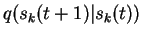must be modified slightly to include the contribution of the previous state value. Saving the notation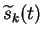for the marginal variance of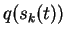, the variance of the conditional distribution is denoted with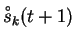. The mean of the distribution,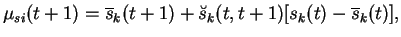(5.45)

depends linearly on the previous state value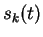. This yields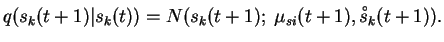(5.46)

The variational parameters of the distribution are thus the mean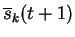, the linear dependence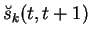and the variance. It should be noted that this dependence is only to the same component of the previous state value. The posterior dependence between the different components is neglected.

The marginal distribution of the states at time instant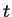may now be evaluated inductively starting from the beginning. Assuming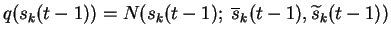, this yields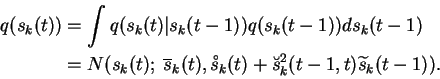(5.47)

Thus the marginal mean is the same as the conditional mean and the marginal variances can be computed using the recursion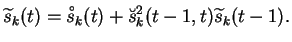(5.48)Next: Combining the two models Up: Bayesian nonlinear state-space model Previous: The prior of the   Contents
Antti Honkela 2001-05-30